Home > A2C > Chapter 2 > Lesson 2.1.1 > Problem2-18

2-18.
1. Perform each operation in part (a) through (d) below. Homework Help ✎

1.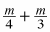2.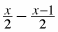3.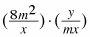4.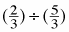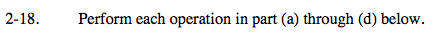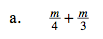Remember to make a common denominator.

$\frac{3\textit{m}}{12}+\frac{4\textit{m}}{12}$

$\frac{7\textit{m}}{12}$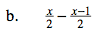Remember to distribute the negative sign.

$\frac{8\textit{ mmy}}{\textit{xmx}}$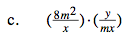This is equivalent to: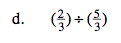Remember division means multiply by the reciprocal of the divisor.

$\left(\frac{2}{3}\right)\cdot\left(\frac{3}{5}\right)$

$\frac{2}{5}$